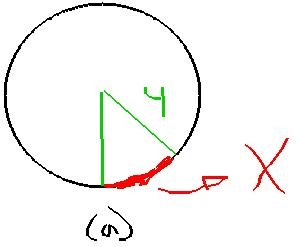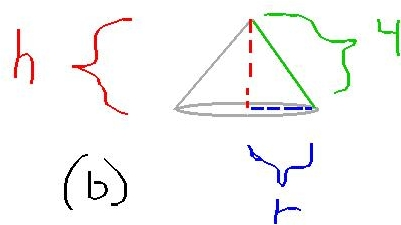Begin with a circular piece of paper with a4-in. radius as shows in (a).sodni3 2020-12-27 Answered

Begin with a circular piece of paper with a4-in. radius as shows in (a). cut out a sector with an arc length of x.Join the two edges of the remaining portion to form a cone with radius r and height h, as shown in (b).a) Explain why the circumference of the bas eofthe cone is $$\displaystyle{8}{\left(\pi\right)}-{x}$$.
b) Express the radius r as a function of x.
c) Express the height h as a function of x.
d) Express the volume V of the cone as afunction of x.

• Questions are typically answered in as fast as 30 minutes

Solve your problem for the price of one coffee

• Math expert for every subject
• Pay only if we can solve it2k1enyvp
a) The circumference of a circle with radius 4 is $$\displaystyle{8}\pi$$. Since the circumference of the base of the cone is equal to the arc length of the remainder of the circle, the conference is $$\displaystyle{8}\pi-{x}$$
b) since r is the radius of the base of the cone, the circuference of that base is equal to $$\displaystyle{2}\pi\cdot{r}$$.
From answer a) that circuference is also equal to $$\displaystyle{8}\pi-{x}$$ so:
$$\displaystyle{8}\pi-{x}={2}\pi{r}$$
$$\displaystyle{r}={\frac{{{8}\pi-{x}}}{{{2}\pi}}}={4}-{\frac{{{x}}}{{{2}\pi}}}$$
c) since h, r and 4 make up a right triangle, we can use the pythagorean theorem:
$$\displaystyle{r}^{{2}}+{h}^{{2}}={16}$$
next we substitute the answer from b) for r and solve for h:
$$\displaystyle{h}=\sqrt{{{16}-{\left({4}-{\frac{{{x}}}{{{2}\pi}}}\right)}^{{2}}}}$$
d) the volume of a cone is:
$$\displaystyle{V}={\frac{{{1}}}{{{3}}}}\pi{r}^{{2}}{h}$$
which means:
$$\displaystyle{V}={\frac{{\pi}}{{{3}}}}{\left({4}-{\frac{{{x}}}{{{2}\pi}}}\right)}^{{2}}\sqrt{{{16}-{\left({4}-{\frac{{{x}}}{{{2}\pi}}}\right)}^{{2}}}}$$